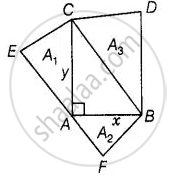# Prove that the area of the equilateral triangle drawn on the hypotenuse of a right angled triangle is equal to the sum of the areas of the equilateral triangles drawn on the other two sides of - Mathematics

Sum

Prove that the area of the equilateral triangle drawn on the hypotenuse of a right-angled triangle is equal to the sum of the areas of the equilateral triangles drawn on the other two sides of the triangle.

#### Solution

Let a right triangle BAC in which ∠A is right angle and AC = y, AB = x.

Three equilateral triangles ΔAEC, ΔAFB and ΔCBD are drawn on the three sides of ΔABC.

Again let area of triangles made on AC, AS and BC are A1, A2 and A3, respectively.To prove: A3 = A1 + A2

Proof: In ΔCAB, by Pythagoras theoren,

BC^2 = AC^2 + AB^2

⇒ BC^2 = y^2 + x^2

⇒ BC = sqrt(y^2 + x^2)

We know that, area of an equilateral triangle = sqrt(3)/2 (Side)2

∴ Area of equilateral ΔAEC, A_1 = sqrt(3)/4 (AC)^2

⇒ A_1 = sqrt(3)/4 y^2   .....(i)

And area of equilateral ΔAFB, A_2 = sqrt(3)/2 (AB)^2

= sqrt(3)/4 sqrt((y^2 + x^2))

= sqrt(3)/4 (y^2 + x^2)

= sqrt(3)/4 y^2 + sqrt(3)/4 x^2

= A1 + A2   ......[From equations (i) and (ii)]

Hence proved.

Concept: Application of Pythagoras Theorem in Acute Angle and Obtuse Angle
Is there an error in this question or solution?

#### APPEARS IN

NCERT Mathematics Exemplar Class 10
Chapter 6 Triangles
Exercise 6.4 | Q 18 | Page 76

Share